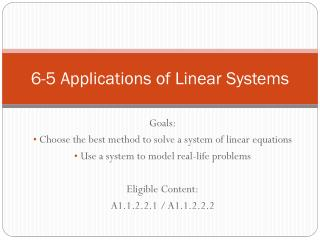DownloadDownload Presentation6-5 Applications of Linear Systems

# 6-5 Applications of Linear Systems

Download Presentation## 6-5 Applications of Linear Systems

- - - - - - - - - - - - - - - - - - - - - - - - - - - E N D - - - - - - - - - - - - - - - - - - - - - - - - - - -
##### Presentation Transcript

1. 6-5 Applications of Linear Systems Goals: Choose the best method to solve a system of linear equations Use a system to model real-life problems Eligible Content: A1.1.2.2.1 / A1.1.2.2.2

2. When to use each method • Graphing • If equations are in slope intercept form • If the problem involves inequalities • If numbers are small and easy to graph • Substitution • If there is a variable without a number in front of it • Linear Combinations • If all variables have numbers in front

3. Which method is best? • 2x + 3y = 4 4x – 2y = 8 • 4x + y = 3 5x + 5y = 16 • y = 2x + 1 y = 3x – 5 • -3x + y = 9 5x + 2y = 10 • y < 7x + 1 y ≥ ½ x – 3 • 5x – 2y = 9 3x + 2y = 7 Substitution Linear Combinations Substitution Graphing Linear Combinations Graphing

4. Word Problems Slope-Intercept Form Standard Form There will be two different situations to compare. You are given a slope and a y-intercept for each situation. Write y = mx + b equations. There will be two different things you are looking for. All of the numbers will fall into two categories. Write Ax + By = C equations.

5. Word Problem #1 You want to burn 380 calories during 40 minutes of exercise. You burn about 8 calories per minute inline skating and 12 calories per minute swimming. How long should you spend doing each activity? Standard Form x = inline skating y = swimming Calories: 8x + 12y = 380 Minutes: x + y = 40 25 minutes inline skating 15 minutes swimming

6. Word Problem #2 Selling frozen yogurt at a fair you make \$565 and use 250 cones. A single-scoop cone costs \$2 and a double-scoop cone costs \$2.50. How many of each type of cone did you sell? Standard Form x = single-scoop y = double-scoop Money: 2x + 2.50y = 565 Cones: x + y = 250 120 single-scoop cones 130 double-scoop cones

7. Word Problem #3 You have a choice of two different Internet service companies. Company A charges \$12 each month plus \$2 per hour. Company B charges \$27 each month plus \$.50 per hour. How many hours would you need to use the Internet for the two companies to be the same? Slope-Intercept Form Company A m = 2 b = 12 y = 2x + 12 Company B m = .50 b = 27 y = .50x + 27 10 hours

8. Word Problem #4 You enroll in a movie club where you earn points to use toward future rentals. Each new release costs \$3 and earns 5 points. Each regular movie costs \$1.50 and earns 3 points. On your recent rental you paid \$12 and earned 22 points. How many of each type of movie did you rent? Standard Form x = new releases y = regular movies Money: 3x + 1.50y = 12 Points: 5x + 3y = 22 2 new releases 4 regular movies

9. Word Problem #5 A retailer offers two options for satellite TV service. A customer may buy the dish for \$150 and then pay \$25 per month for service. The other option is to rent the dish for free and pay \$35 per month for service. After how many months will the two companies be the same? Slope-Intercept Form Company A m = 25 b = 150 y = 25x + 150 Company B m = 35 b = 0 y = 35x + 0 15 months

10. FUNDRAISINGFor a school fundraiser, Marcus and Anisa participated in a walk-a-thon. In the morning, Marcus walked 11 miles and Anisa walked 13. Together they raised \$523.50. After lunch, Marcus walked 14 miles and Anisa walked 13. In the afternoon they raised \$586.50. How much did each raise per mile of the walk-a-thon? A. Marcus: \$22.00, Anisa: \$21.65 B. Marcus: \$21.00, Anisa: \$22.50 C. Marcus: \$24.00, Anisa: \$20.00 D. Marcus: \$20.75, Anisa: \$22.75

11. Practice • Page 367 #5

12. Homework • Worksheet – “6-5 Applications of Linear Systems Homework #1”